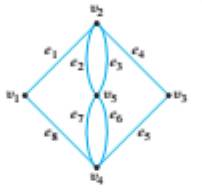Chapter 10.1, Problem 12ES### Discrete Mathematics With Applicat...

5th Edition
EPP + 1 other
ISBN: 9781337694193

#### Solutions

Chapter
Section### Discrete Mathematics With Applicat...

5th Edition
EPP + 1 other
ISBN: 9781337694193
Textbook Problem
1 views

# Determine which of the graph in 12-17 have Euler circuits. If the graph does not have an Euler circuit, explain why not. If it does have an Euler circuit, describe one.To determine

Determine which graph have Euler circuits. If the graph does not have an Euler circuit, explain why not. If it does have an Euler circuit, describe one.

Explanation

Given information:

Calculation:

We know that: the degree of a vertex is the number of the edges that connect to the vertex.

A loop at a vertex counts as two edges.

An Euler circuit is a circuit that contains every edge of the graph.

A connected graph has an Euler circuit if and only if each of the vertices has an even degree.

The graph is connected, because there is a walk between every pair of vertices.

Let us next determine the degree of every vertex, which is the number of edges that connect to the vertex.

deg(v1)=2deg(v2)=4deg(

### Still sussing out bartleby?

Check out a sample textbook solution.

See a sample solution

#### The Solution to Your Study Problems

Bartleby provides explanations to thousands of textbook problems written by our experts, many with advanced degrees!

Get Started